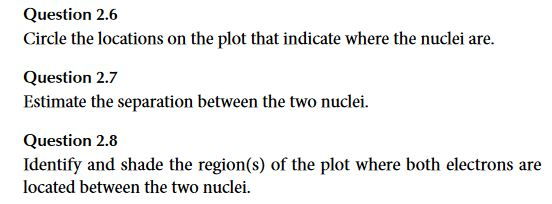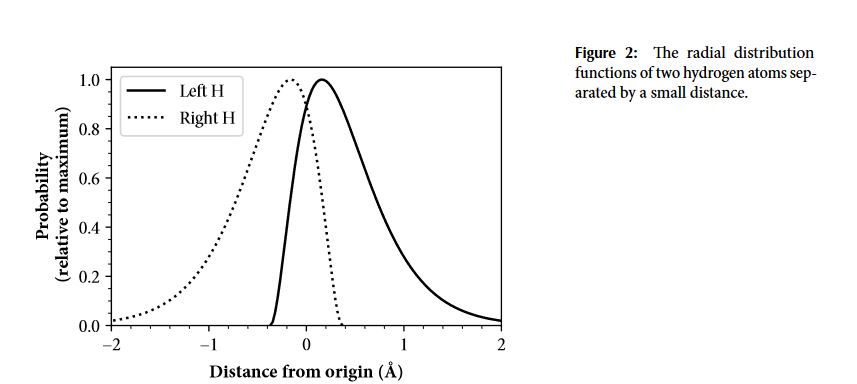# Question 2.6 Circle the locations on the plot that indicate where the nuclei are. Question 2.7...

###### Question:Question 2.6 Circle the locations on the plot that indicate where the nuclei are. Question 2.7 Estimate the separation between the two nuclei. Question 2.8 Identify and shade the region(s) of the plot where both electrons are located between the two nuclei.
Figure 2: The radial distribution functions of two hydrogen atoms sep- arated by a small distance. Left H ...... Right H Probability (relative to maximum) - 2 Distance from origin (Å)

#### Similar Solved Questions

##### Solve for t. -0.025t N=Nge (used in chemistry)
Solve for t. -0.025t N=Nge (used in chemistry)...